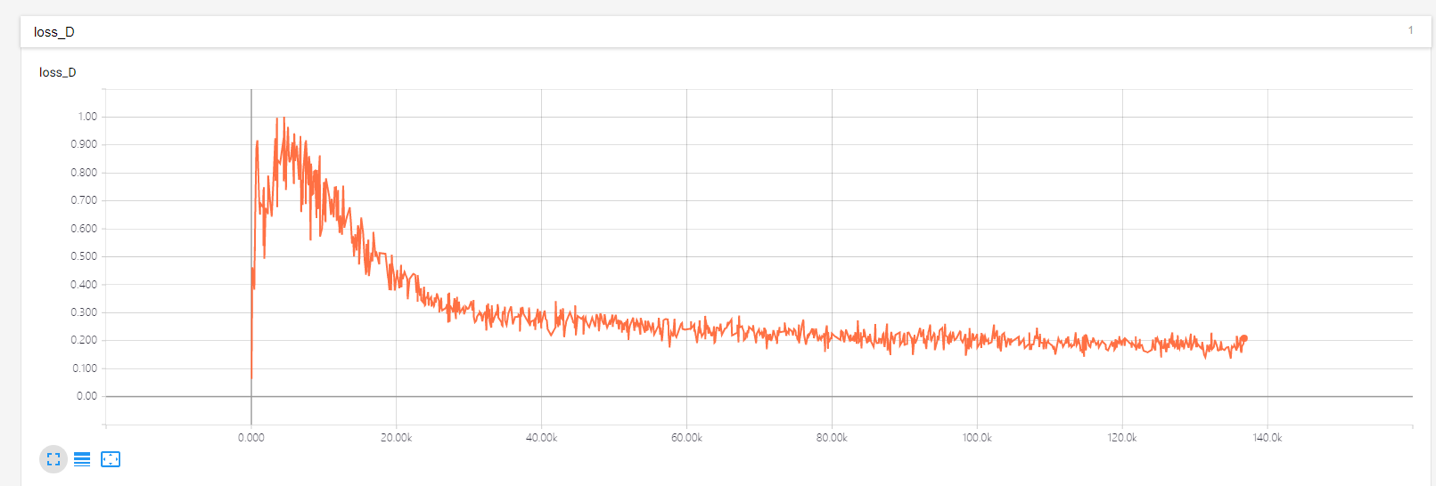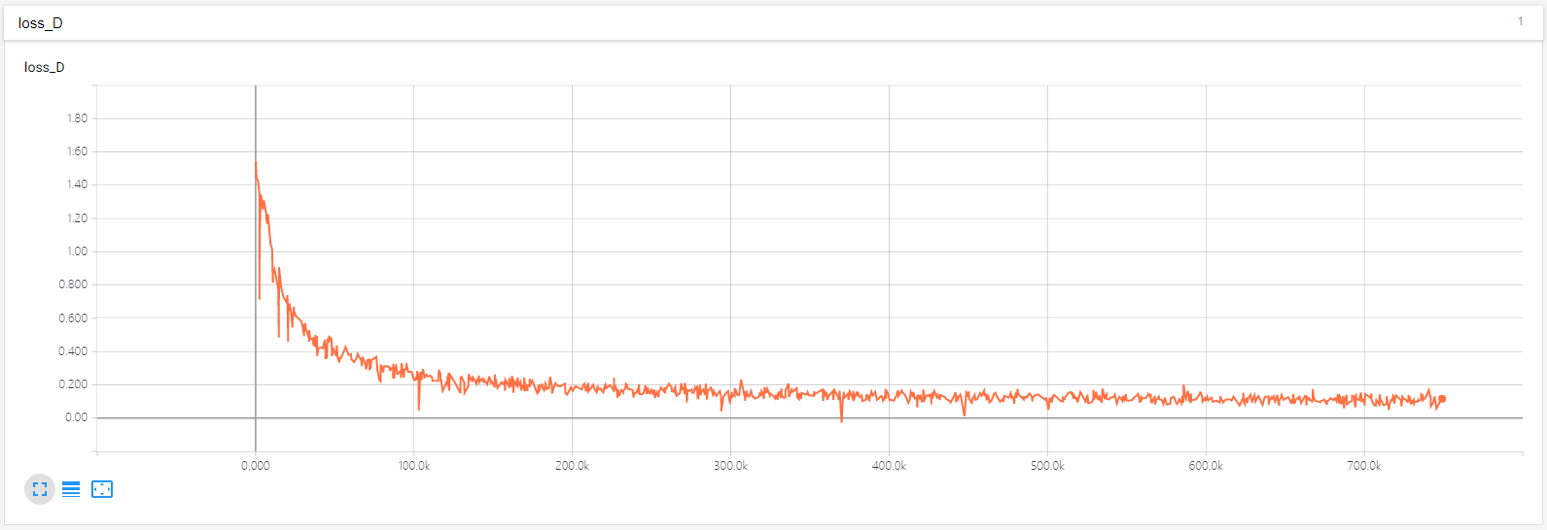## Wgan介绍

Wgan是在Dcgan的基础上，使用wasserstein距离替代KL距离。使用wasserstein距离的好处是，即使当两个概率分布是没有重合的时候，同样能衡量出一个距离，而这种情况下，dcgan中使用的KL距离是会为0，导致梯度消失，训练过程不稳定。 而如果要让discremator能够衡量出wasserstein距离，需要让discremator满足Leibniz连续。

## 关键细节

### Weight clip

for p in netD.collect_params():
param = netD.collect_params(p)[p]
param.set_data(mx.nd.clip(param.data(), opt.clamp_lower, opt.clamp_upper))


### Weight的初始化

for layer in layers:
classname = layer.__class__.__name__
if classname.find('Conv') != -1:
layer.weight.set_data(mx.ndarray.random.normal(0.0,0.02,shape=layer.weight.data().shape))
elif classname.find('BatchNorm') != -1:
layer.gamma.set_data(mx.ndarray.random.normal(1.0, 0.02,shape=layer.gamma.data().shape))
layer.beta.set_data(mx.ndarray.zeros(shape=layer.beta.data().shape))


### 训练discremator

discremator的最后输出是没有sigmoid的

#真实样本计算出一个
errD_real = netD(data)
#随机噪声生成出一个图像
fake = netG(noise)
#detach操作可以减少计算量，梯度无需传递到generator
errD_fake = netD(fake.detach())
#计算损失函数
errD = errD_real - errD_fake


### 训练generator

#从随机噪声中生成一个图像
fake = netG(noise)
errG = netD(fake)
#计算损失函数
errG.backward()


### Rmsprop

$\epsilon$只是一个防止分母为0的值，通常取默认值$10^{-8}$,因为这个值非常小，一般放在根号里面和外面影响不大，但因为在wgan中，$g$一般也比较小，所以在$\epsilon$就对结果产生比较大的影响了，当把$-loss D$画出来之后可以看到前面部分出现了训练不稳定的现象。 解决方法是调小$\epsilon$的值，将generator的$\epsilon$参数设置为$10^{-14}$，将discremator的$\epsilon$参数设置为$10^{-12}$，最后 训练结果如下